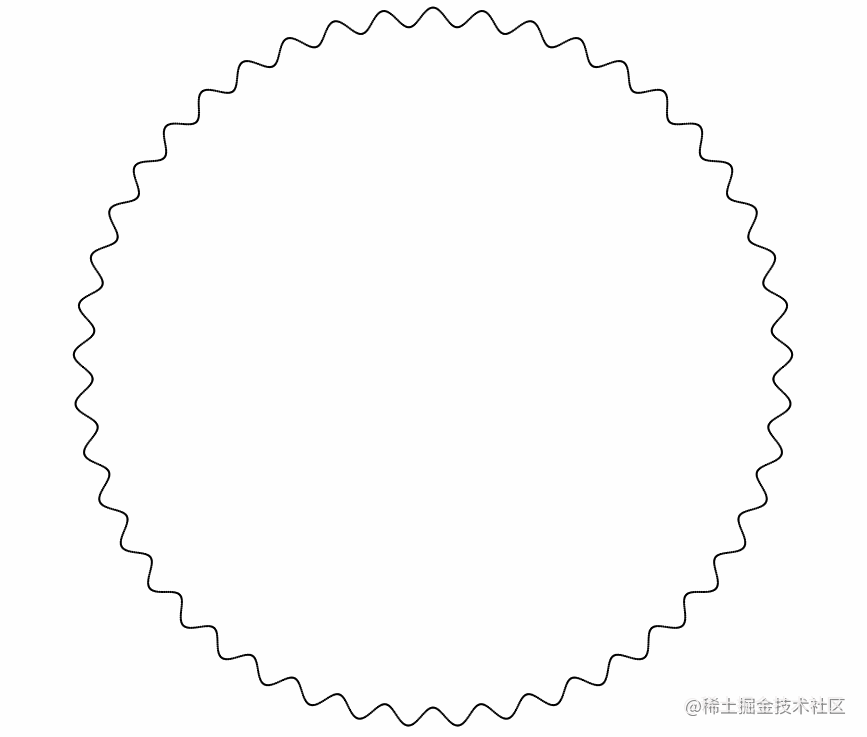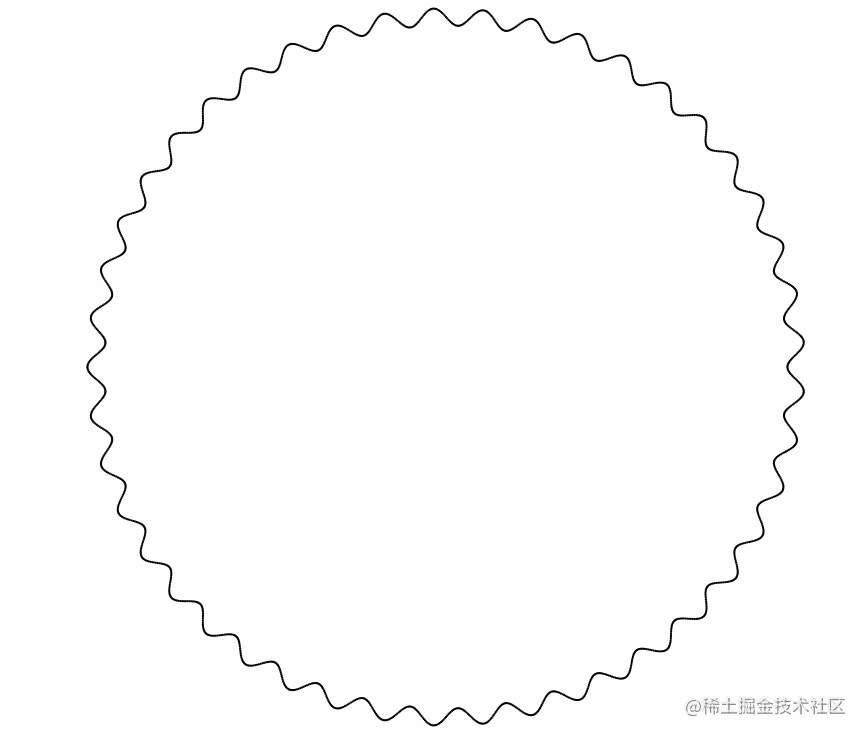# 為了學會更多炫酷的 canvas 效果，我熬夜複習了三角函式相關的知識點

theme: scrolls-light highlight: atom-one-dark

### 弧度與角度之間的互相轉換

let angle //角度

radian = angle * Math.PI / 180

angle = radian / Math.PI * 180

### 正弦曲線

``html` 中只有一個 `canvas` 標籤；`css` 也很簡單，如下：`css {margin: 0; padding: 0;} body { display: flex; justify-content: center; align-items: center; width: 100%; height: 100vh; overflow: hidden; } `就這幾行樣式，我想大家應該都已經很熟悉了，接下來 `JS` 才是我們的重點，這裡依舊採用面向物件的方式來編寫程式碼，這樣讓我們的程式碼看起來更有條理性，首先還是相關的基礎準備程式碼，如下：`js class SineAnimate { constructor() { / @type {HTMLCanvasElement} / this.canvas = document.getElementById('canvas'); this.ctx = this.canvas.getContext('2d'); this.canvas.width = 800; this.canvas.height = 800; this.dots = []; } } ````` 前面幾行就是基本的獲取```canvas`以及`ctx`，並設定`canvas` 的寬和高，最後一行主要是用於存放我們後續生成的點，這裡用一個個小點來畫出正弦效果。

``````    this.init();
}

init() {
for (let i = 0; i < this.canvas.width; i++) {
this.dots.push(new Dots(i, this.canvas, this.ctx));
}

this.draw();
}

draw() {
this.ctx.clearRect(0, 0, this.canvas.width, this.canvas.height);
for (const key in this.dots) {
let dot = this.dots[key];
dot.update();
dot.draw();
}
}
``````

}

new SineAnimate(); ````` 在```SineAnimate`類中，定義了一個`init`方法，並通過當前`canvas`的寬度動態生成了 **800** 個點，然後還定義了一個`draw`方法，在這個方法中將前面生成的 **800** 個點渲染在頁面中，最後不要忘記例項化`SineAnimate` 類，最終實現的正弦效果如下圖所示：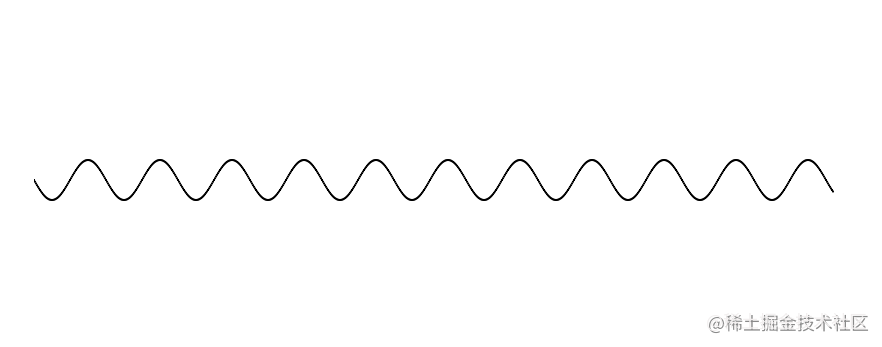``````draw() {
this.ctx.clearRect(0, 0, this.canvas.width, this.canvas.height);
this.ctx.fillRect(0, this.canvas.height / 2, this.canvas.width, 1);
for (const key in this.dots) {
let dot = this.dots[key];
dot.update();
dot.draw();
}
}
``````

} ````` 在```SineAnimate`類的`draw`方法中通過`canvas`的`fillRect` 方法，繪製一條輔助線，實現出來的效果如下所示：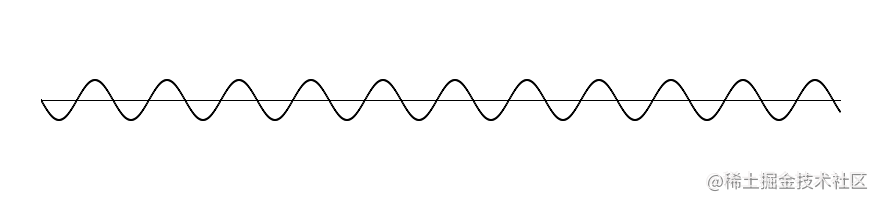``````draw() {
//...other code
this.ctx.fillRect(this.x, this.y, 1, this.canvas.height / 2 - this.y);
}
``````

} ````` 在```Dots`類的`draw`方法中，通過`fillRect` 方法來繪製點到垂直距離的線段，實現的效果如下所示：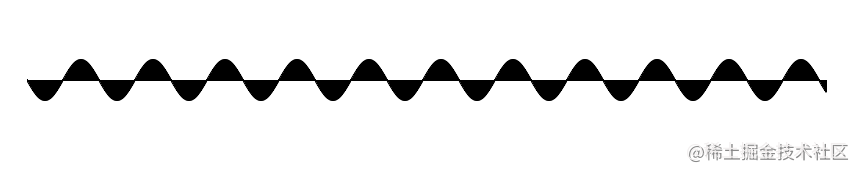### 環繞圓心的正弦曲線

``````init() {
for (let i = 0; i < 360; i++) {
this.dots.push(new Dots(i, this.canvas, this.ctx));
}

this.draw();
}
``````

} `修改完 `SineAnimate` 類，接下來就需要修改 `Dots` 類了，讓我們一起來看相關的程式碼，如下：`js class Dots { constructor(d, canvas, ctx) { this.d = d; this.x = 0; this.y = 0; this.r = 0; this.w = 0; this.canvas = canvas; this.ctx = ctx; } update() { this.r = this.w * Math.sin(this.d * Math.PI / 4) + 350; this.x = this.r * Math.cos(this.d * Math.PI / 180) + this.canvas.width / 2; this.y = this.r * Math.sin(this.d * Math.PI / 180) + this.canvas.height / 2; } draw() { this.ctx.beginPath(); this.ctx.arc(this.x, this.y, 1, 0, Math.PI * 2, 0); this.ctx.fill(); } } ````` 在```Dots`類中，重新定義了圓的半徑和寬度，然後在`update` 方法中，按照 360 度的角度來生成點，根據上面正弦曲線公式生成不同角度下的半徑值，然後再根據最前面的知識點，通過圓的半徑與角度得到最終確定的座標點，最終實現了將所有的點連線成一個圓，如下圖所示：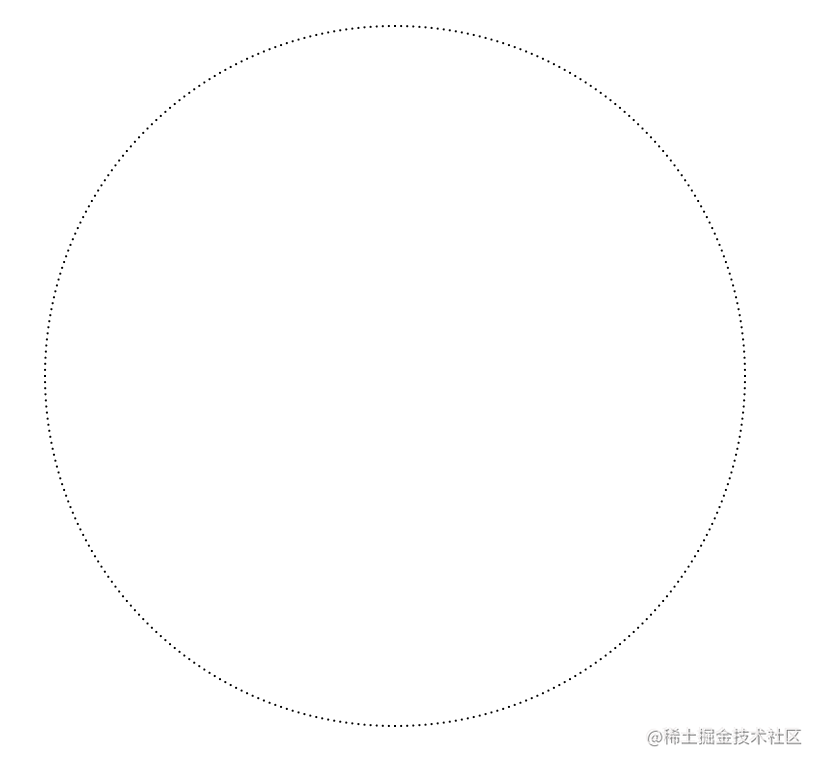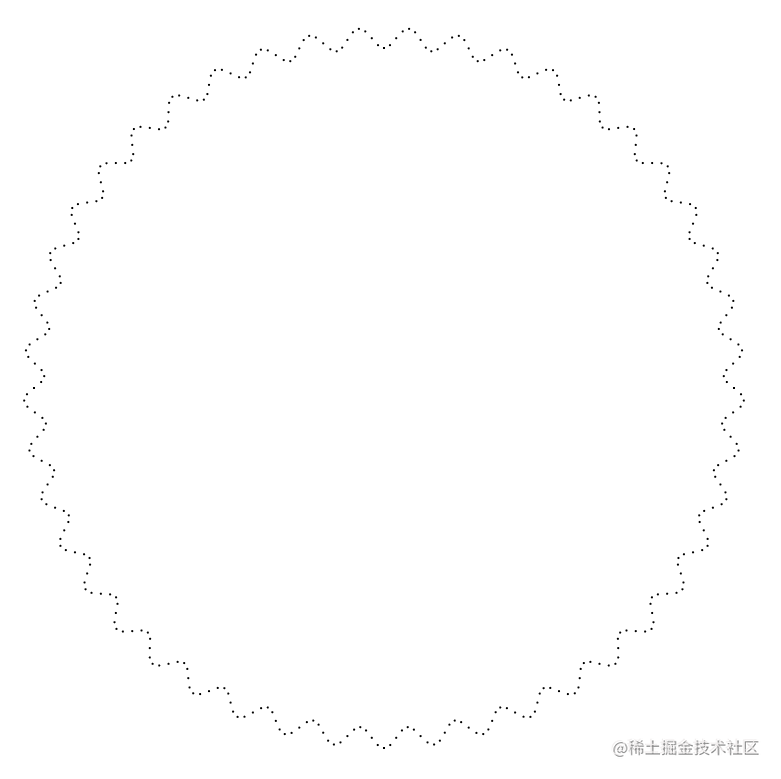``````init() {
for (let i = 0; i < 360; i += 0.2) {
this.dots.push(new Dots(i, this.canvas, this.ctx));
}
}

...other code
``````

} ````` 修改```init` 中迴圈的最後一個引數，實現出來的效果如下所示：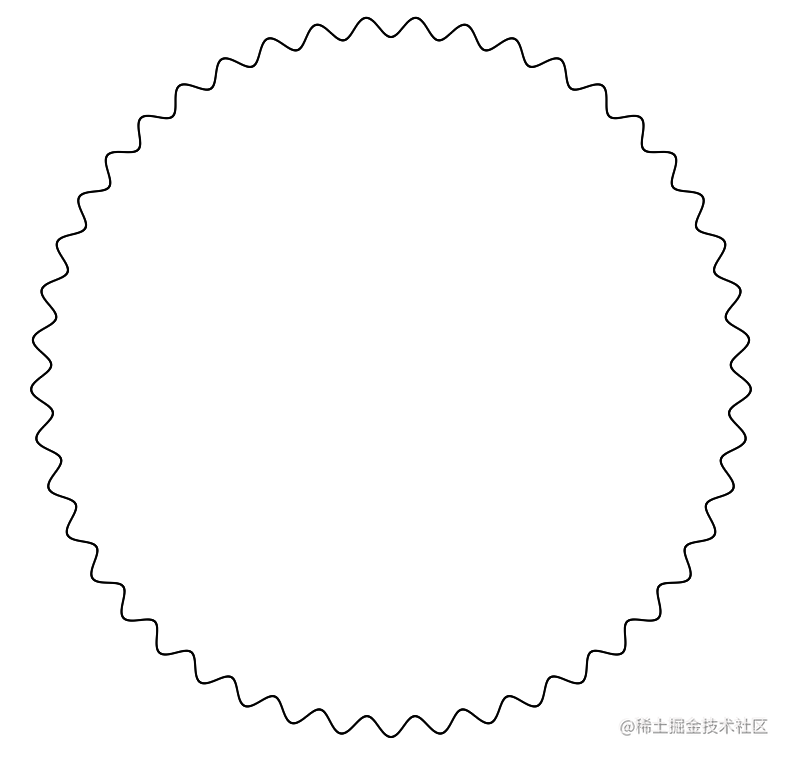``````draw() {
this.ctx.clearRect(0, 0, this.canvas.width, this.canvas.height);
this.ctx.beginPath();
this.ctx.arc(this.canvas.width/2, this.canvas.height/2, 350, 0, Math.PI * 2, 0);
this.ctx.stroke();
for (const key in this.dots) {
let dot = this.dots[key];
dot.update();
dot.draw();
}
}
``````

} ``` 跟前面的正弦輔助線一樣的，這裡只是畫在圓上，實現的效果如下圖所示：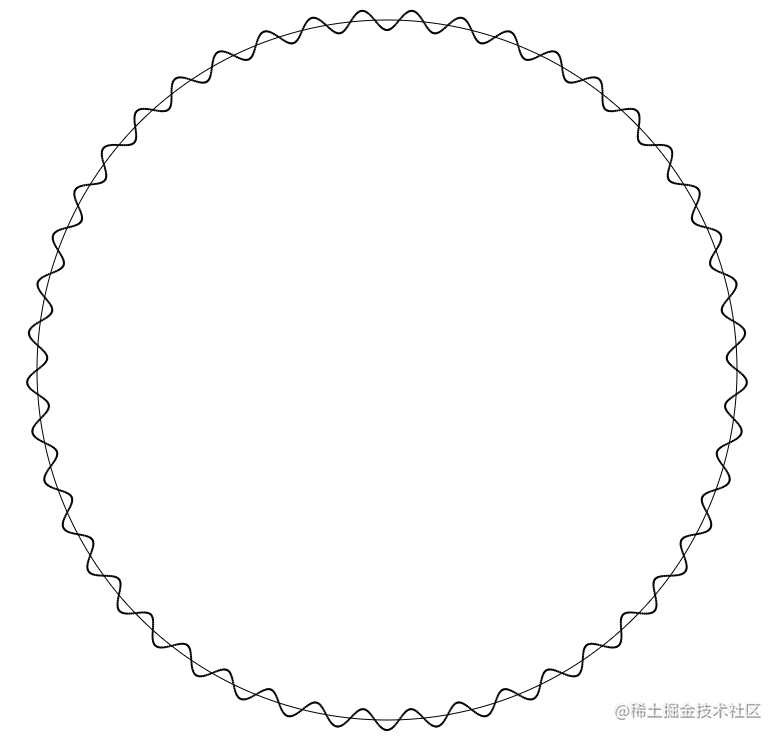### 動態環繞圓心的正弦曲線